# C++ Core Guidelines: Avoid Bounds Errors

When you access an element outside a container of the STL, the result is not so promising. Your effect may be an error or undefined behavior. Undefined behavior means all bets are open.First of all: What is a bounds error? A bounds error happens when you read or write beyond the elements of a container. The result is different depending on the used container. Of course, the C++ core guidelines are very concrete.

### SL.con.3: Avoid bounds errors

The C++ core guidelines start with a bad example using unsafe C-functions to fill and compare a std::array.

```std::array<int, 10> a, b;
std::memset(a.data(), 0, 10);         // BAD, and contains a length error (length = 10 * sizeof(int))
std::memcmp(a.data(), b.data(), 10);  // BAD, and contains a length error (length = 10 * sizeof(int))
```

The comments to the code already say it. The length of the C-arrays is not 10 but 10 * sizeof(int). The solution is obvious. Use the functionality of the std::array.

##Modernes C++ Mentoring

Be part of my mentoring programs:

• "Fundamentals for C++ Professionals" (open)
• "Design Patterns and Architectural Patterns with C++" (open)
• "C++20: Get the Details" (reopens December 2023)
• Do you want to stay informed about my mentoring programs: Subscribe via E-Mail.

```std::array<int, 10> a;
std::array<int, 10> b;

std::array<int, 10> c{};

a.fill(0);                         // (1)
std::fill(b.begin(), b.end(), 0);  // (2)

if ( a == b ){                     // (3)
// ...
}
```

In this case, the std::array a and b are not initialized. On the opposite, all values of care are initialized to 0. Line (1) sets all values of a to 0, and line 2 uses the function templates std::fill. Comparison is also quite convenient (line 3).

Using a container outsides its range is, in general, undefined behavior. Let me see what that means.

### Bounds Errors

The most elementary sequential container we have in C++ is the C-array.

### C-Array

The effect of an overflow or an underflow is the same: memory corruption and undefined behavior. Let’s make a simple test with an int array. How long will the next program run?

```// overUnderflow.cpp

#include <cstddef>
#include <iostream>

int main(){

int a;
int n{};

while (true){
if (!(n % 100)){
std::cout << "a[" << n << "] = " << a[n] << ", a[" << -n << "] = " << a[-n] << "\n";
}
a[n] = n;
a[-n] = -n;
++n;
}

}
```

Way too long! The program writes each 100th array entry to std::cout.Okay, what will happen if I use a sequential container from the STL? Here we are:

### Sequential Containers of the STL

The index operator is available for std::array, std::vector, std::deque, and std::string. For simplicity reasons, I count a std::string as a sequential container. This means all containers support random access and return a random access iterator. To bore you not to death, I use only a std::array and a std::vector in my next experiment.

#### std::array

This is the modified program for std::array:

```// overUnderflowStdArray.cpp

#include <array>
#include <iostream>

int main(){

std::array<int, 1> a;
int n{};

while (true){
if (!(n % 100)){
std::cout << "a[" << n << "] = " << a[n] <<
", a[" << -n << "] = " << a[-n] << "\n";
}
a[n] = n;
a[-n] = -n;
++n;
}

}
```

Using the index operator for a C++ array is not better than using it for a C array.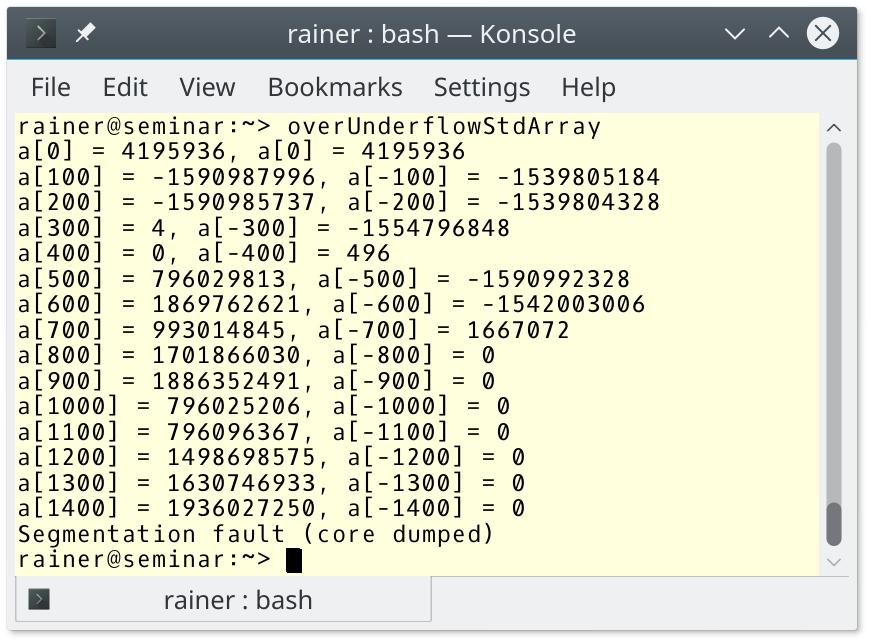Maybe, a std::vector comes to our rescue.

#### std::vector

```// overUnderflowStdVector.cpp

#include <vector>
#include <iostream>

int main(){

std::vector<int> a{1};
int n{};

while (true){
if (!(n % 100)){
std::cout << "a[" << n << "] = " << a[n] <<
", a[" << -n << "] = " << a[-n] << "\n";
}
a[n] = n;
a[-n] = -n;
++n;
}

}
```

Because the std::vector creates its objects on the heap and not on the stack, such as the C- and C++-array, it takes quite a while for the program to fail. The screenshots show the beginning and the end of the under- and overflow.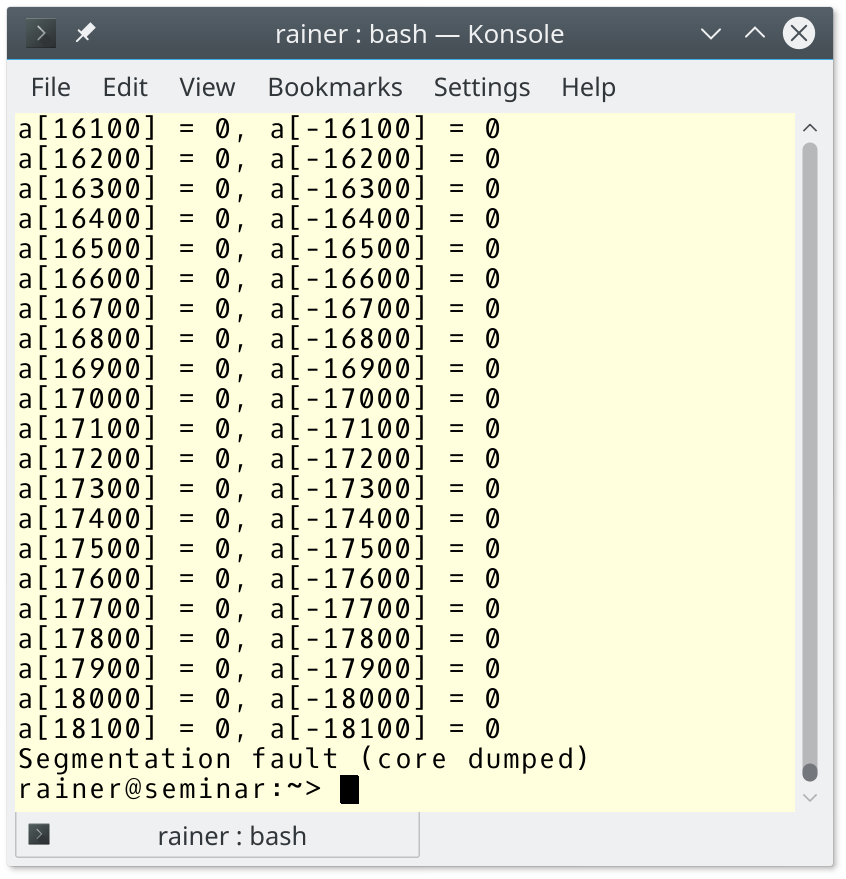Additionally, associative containers such as std::map and std::unordered_map also support the index operator.

### Associative Containers of the STL

What happens when you use a non-existing key in a std::map or std::unordered_map?

```// indexOperatorMapAndUnorderedMap.cpp

#include <iostream>
#include <map>
#include <unordered_map>
#include <string>

int main(){

std::cout << std::boolalpha << std::endl;

std::map<std::string, int> myMap;
std::unordered_map<std::string, bool> myUnorderedMap;

std::cout << "myMap[DoesNotExist]: " << myMap["DoesNotExist"] << std::endl;

std::cout << "myUnorderedMap[DoesNotExist]: " << myUnorderedMap["DoesNotExist"] << std::endl;

}
```

In the case of the associative container, the value you get is well-defined if the key is unavailable. The value must be DefaultConstructible because the default constructor is invoked if the key is unavailable. This creates der literal 0 in the first case and the literal false in the second case.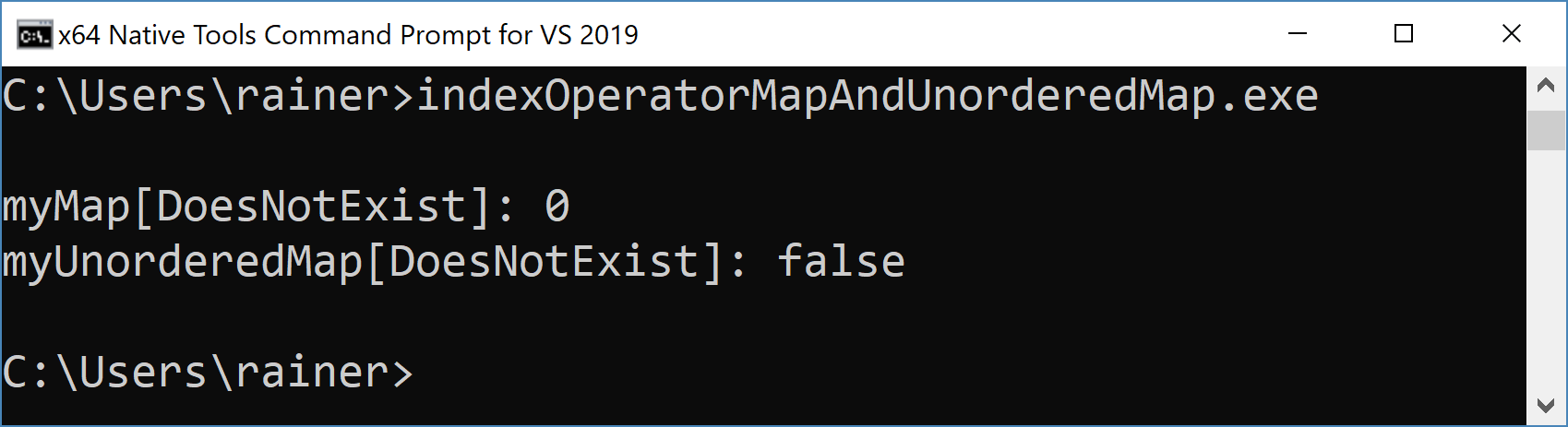Okay, the essential question of the guideline remains: How can you avoid bounds errors?

### Avoid bounds errors

In the case of the C-array, there is no rescue to detect a bounds error. For the C++ containers, including std::string, there is a method at which checks the bounds. All C++ container throws a std::out_of_range exception if you access a non-existing element. The std::string shows this impressive.

```// stringBoundsCheck.cpp

#include <stdexcept>
#include <iostream>
#include <string>

int main(){

std::cout << std::endl;

std::string str("1123456789");

str.at(0) = '0';                                   // (1)

std::cout << str << std::endl;

std::cout << "str.size(): " << str.size() << '\n';
std::cout << "str.capacity() = " << str.capacity() << '\n';

try {
str.at(12) = 'X';                              // (2)
}
catch (const std::out_of_range& exc) {
std::cout << exc.what() << std::endl;
}

std::cout << std::endl;

}

```

Setting the first character of the string str to ‘0’ (line 1) is fine, but accessing a character outside the size is an error. This even holds if the access is within the capacity but outside the size of the std::string.

1. The size of a std::string is the number of elements the std::string has.
2. The capacity of a std::string is the number of elements a std::string could have without allocating additional memory.

The error message of the windows compiler 19.20 is unspecific.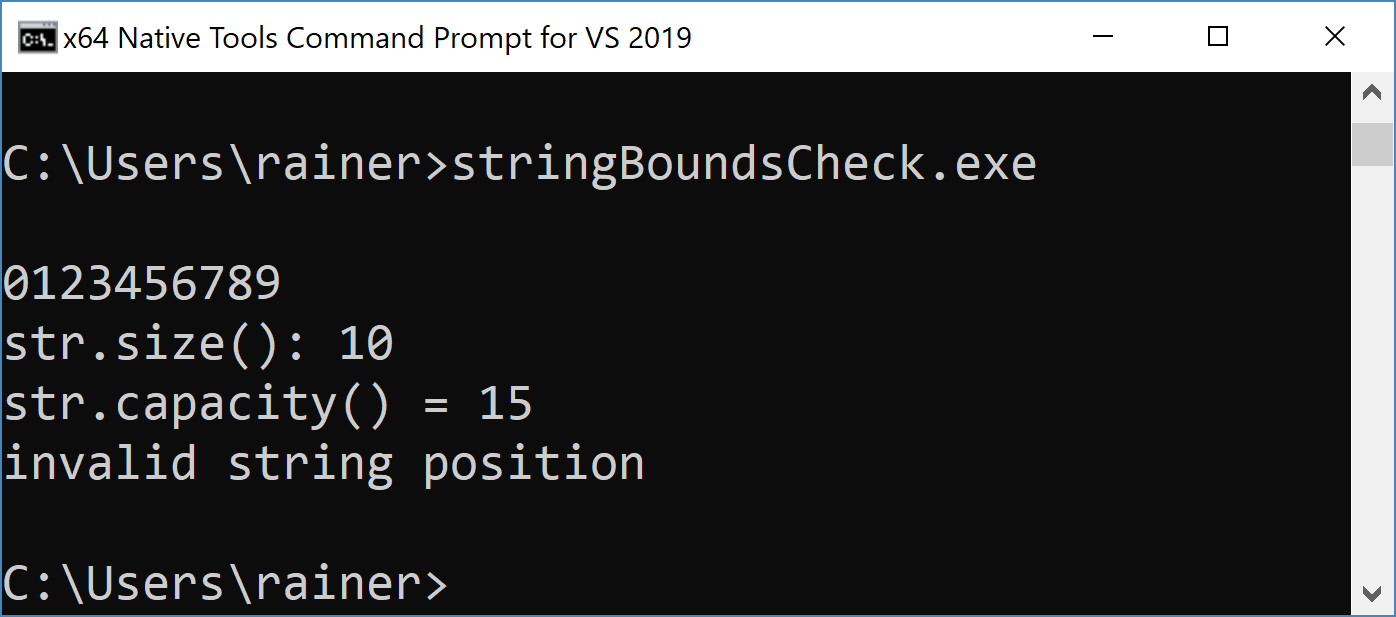The error message for GCC 8.2 is quite specific.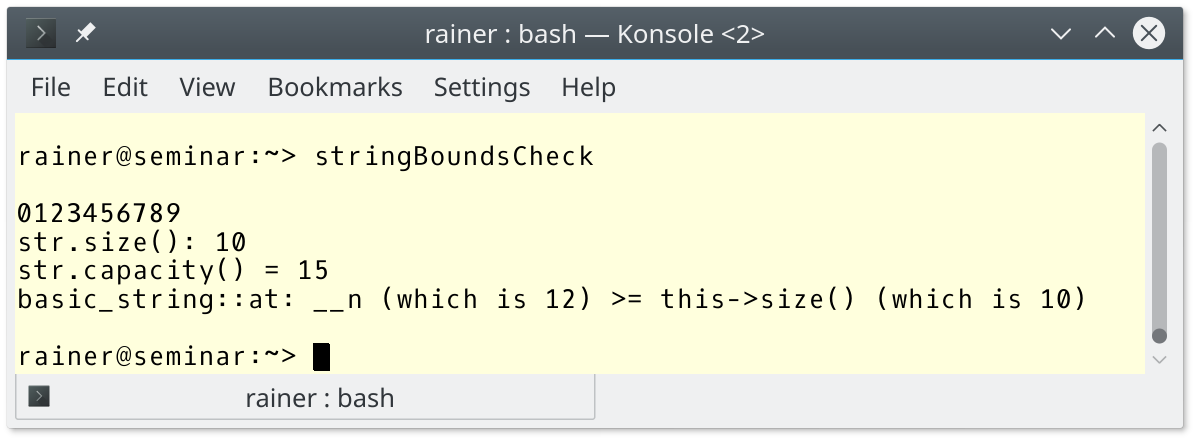## What’s next?

This post was the last one to the containers of the STL. The next post is about the various string types.

Thanks a lot to my Patreon Supporters: Matt Braun, Roman Postanciuc, Tobias Zindl, G Prvulovic, Reinhold Dröge, Abernitzke, Frank Grimm, Sakib, Broeserl, António Pina, Sergey Agafyin, Андрей Бурмистров, Jake, GS, Lawton Shoemake, Jozo Leko, John Breland, Venkat Nandam, Jose Francisco, Douglas Tinkham, Kuchlong Kuchlong, Robert Blanch, Truels Wissneth, Kris Kafka, Mario Luoni, Friedrich Huber, lennonli, Pramod Tikare Muralidhara, Peter Ware, Daniel Hufschläger, Alessandro Pezzato, Bob Perry, Satish Vangipuram, Andi Ireland, Richard Ohnemus, Michael Dunsky, Leo Goodstadt, John Wiederhirn, Yacob Cohen-Arazi, Florian Tischler, Robin Furness, Michael Young, Holger Detering, Bernd Mühlhaus, Matthieu Bolt, Stephen Kelley, Kyle Dean, Tusar Palauri, Dmitry Farberov, Juan Dent, George Liao, Daniel Ceperley, Jon T Hess, Stephen Totten, Wolfgang Fütterer, Matthias Grün, Phillip Diekmann, Ben Atakora, Ann Shatoff, Rob North, Bhavith C Achar, and Marco Parri Empoli.

Thanks, in particular, to Jon Hess, Lakshman, Christian Wittenhorst, Sherhy Pyton, Dendi Suhubdy, Sudhakar Belagurusamy, Richard Sargeant, Rusty Fleming, John Nebel, Mipko, Alicja Kaminska, Slavko Radman, and David Poole.

 My special thanks to EmbarcaderoMy special thanks to PVS-StudioMy special thanks to Tipi.buildMy special thanks to Take Up Code## Seminars

I’m happy to give online seminars or face-to-face seminars worldwide. Please call me if you have any questions.

### Standard Seminars (English/German)

Here is a compilation of my standard seminars. These seminars are only meant to give you a first orientation.

• C++ – The Core Language
• C++ – The Standard Library
• C++ – Compact
• C++11 and C++14
• Concurrency with Modern C++
• Design Pattern and Architectural Pattern with C++
• Embedded Programming with Modern C++
• Generic Programming (Templates) with C++

#### New

• Clean Code with Modern C++
• C++20

### Contact Me

Modernes C++ Mentoring,0 replies